Ex 10.3

Chapter 10 Class 11 Straight Lines (Term 1)
Serial order wise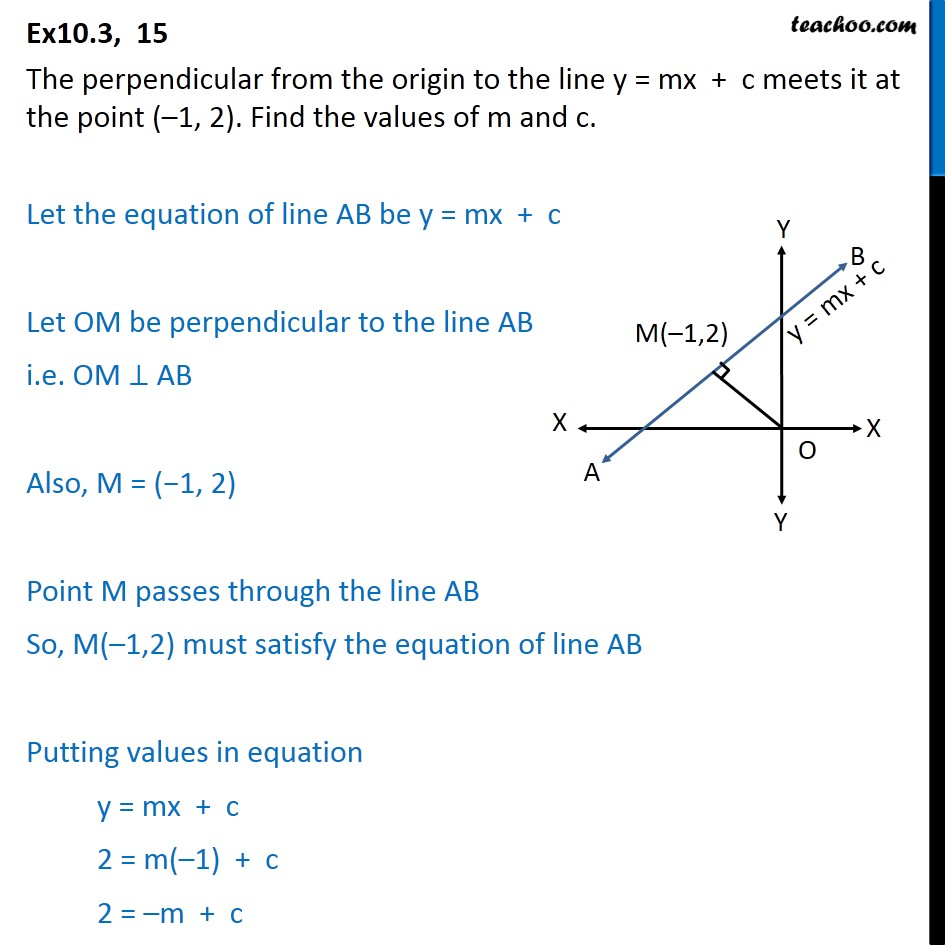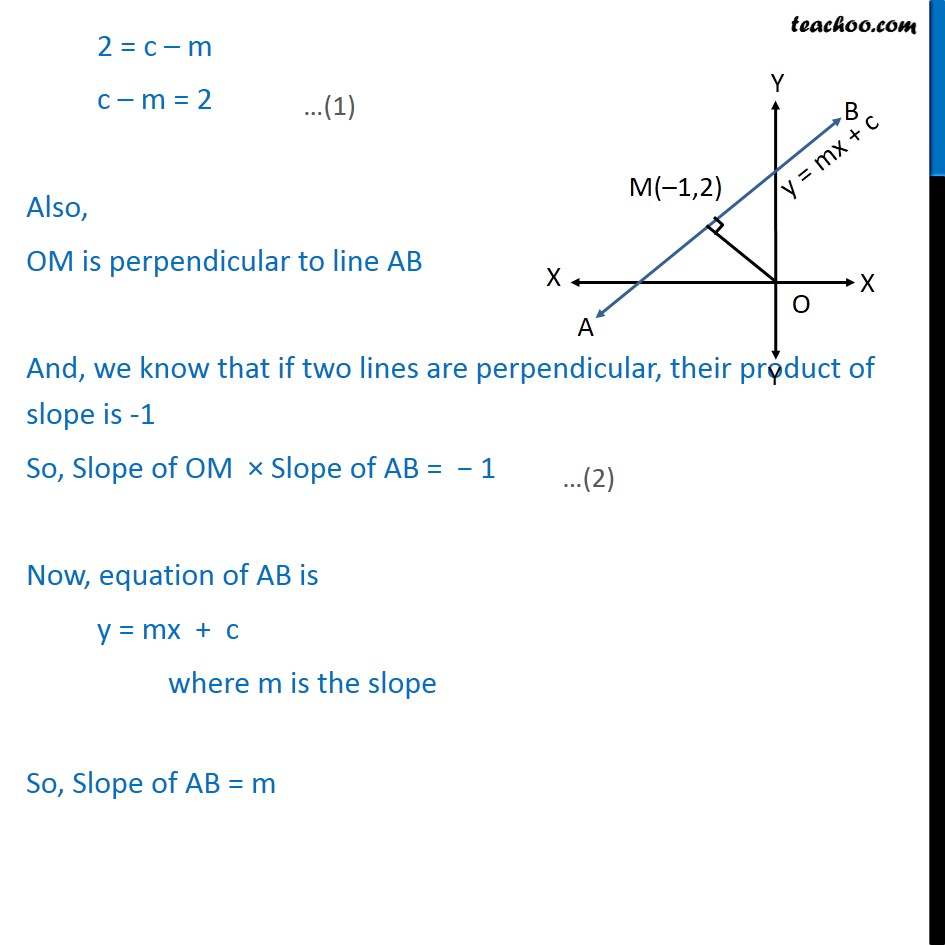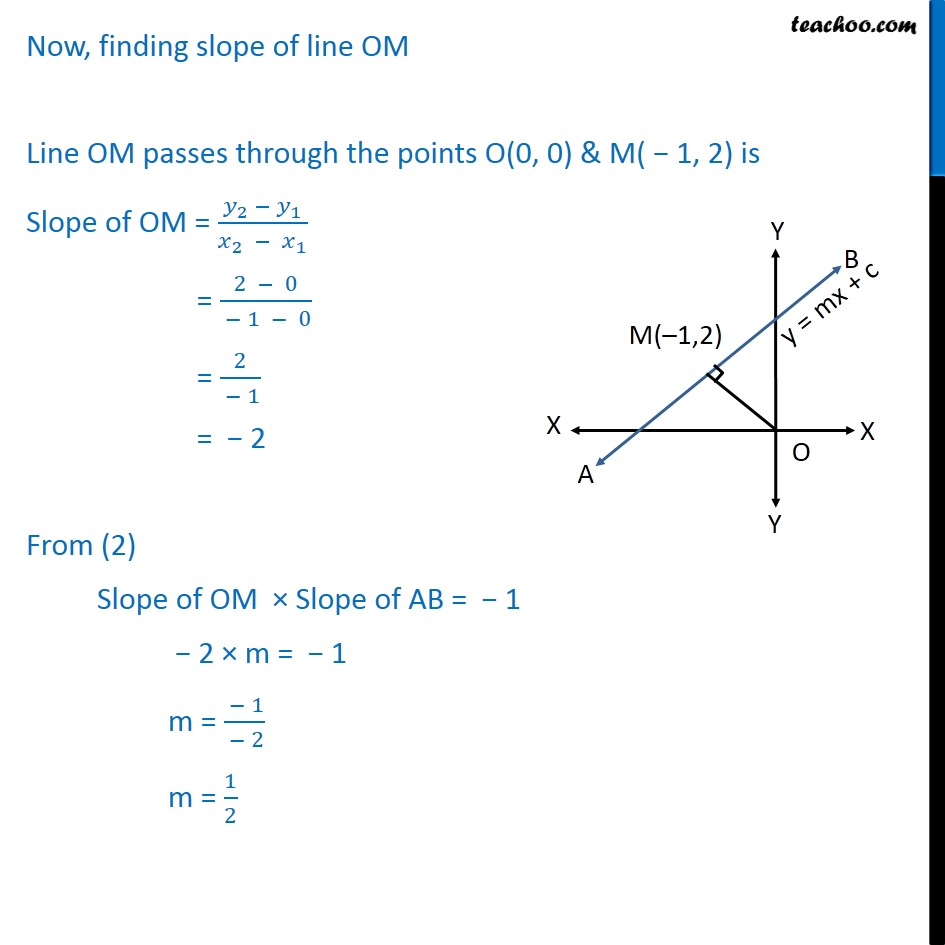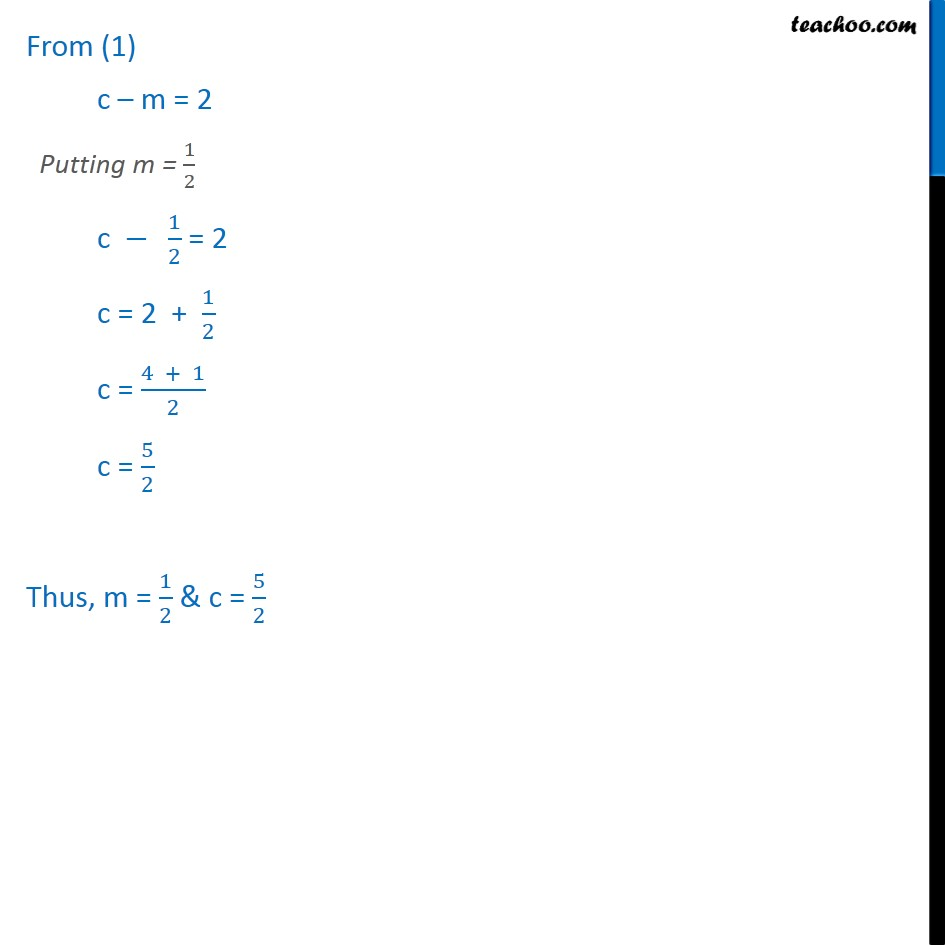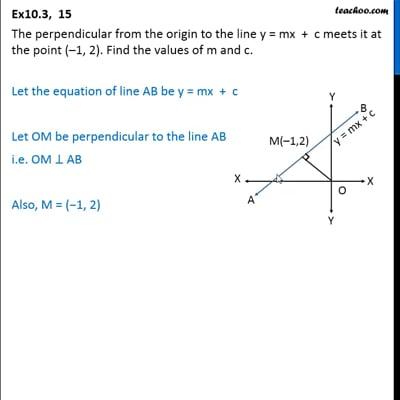This video is only available for Teachoo black users

### Transcript

Ex10.3, 15 The perpendicular from the origin to the line y = mx + c meets it at the point (–1, 2). Find the values of m and c. Let the equation of line AB be y = mx + c Let OM be perpendicular to the line AB i.e. OM ⊥ AB Also, M = (−1, 2) Point M passes through the line AB So, M(–1,2) must satisfy the equation of line AB Putting values in equation y = mx + c 2 = m(–1) + c 2 = –m + c 2 = c – m c – m = 2 Also, OM is perpendicular to line AB And, we know that if two lines are perpendicular, their product of slope is -1 So, Slope of OM × Slope of AB = − 1 Now, equation of AB is y = mx + c where m is the slope So, Slope of AB = m Now, finding slope of line OM Line OM passes through the points O(0, 0) & M( − 1, 2) is Slope of OM = (𝑦_2 − 𝑦_1)/(𝑥_2 − 𝑥_1 ) = (2 − 0)/( − 1 − 0) = 2/( − 1) = − 2 From (2) Slope of OM × Slope of AB = − 1 − 2 × m = − 1 m = ( − 1)/( − 2) m = 1/2 From (1) c – m = 2 Putting m = 1/2 c − 1/2 = 2 c = 2 + 1/2 c = (4 + 1)/2 c = 5/2 Thus, m = 1/2 & c = 5/2